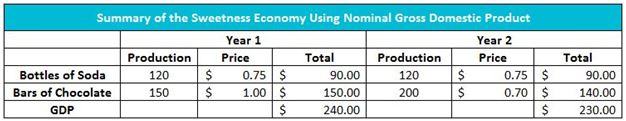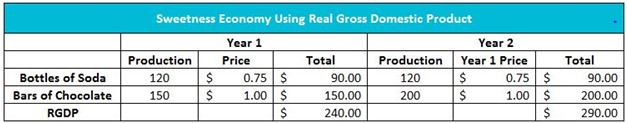# GDP Deflator

## Definition of GDP Deflator:

The GDP deflator is used to convert the nominal gross domestic product to the real gross domestic product.

## Detailed Explanation:

Economists use the GDP deflator to determine what portion of the increase in nominal GDP is caused by a change in production and what portion is caused by a change in prices. It is a “deflator” because generally, prices increase, but the same formula would be used in a period of deflation.

Nominal GDPx 100 = GDP Deflator
Real GDP

Sweetness is a tiny country that produces only soft drinks and chocolate. In Year 1, the economy produced 120 bottles of soft drinks and 150 bars of chocolate. The price of a bottle of soft drink was \$0.75, and the price of a bar of chocolate was \$1.00. Sweetness’s GDP equaled \$240 in Year 1. This is calculated by adding the market value of the soft drinks produced (120 x \$0.75 = \$90) and the market value of chocolate produced (150 x \$1.00 = \$150).

Assume the cost of producing chocolate bars is less in Year 2. The forces of supply and demand decreased the price of a bar to \$0.70. The quantity demanded increased to 200 bars at \$0.70. To meet the added demand, production was increased by 33 percent to 200 bars. Therefore, Sweetness’s GDP decreased to \$230 in Year 2 even though production of chocolate bars increased and the production of soda remained unchanged. The table below summarizes the calculation of nominal GDP in Sweetness in Years 1 and 2.Even though the actual production of goods increases in Year 2, the lower price of chocolate has a greater impact on GDP than the increase in production. To measure the real increase in production, economists hold the price of the goods and services constant. The table below shows that the real gross domestic product increases to \$290 when using Year 1 prices. The real gross domestic product (RGDP) is the gross domestic product adjusted for changes in prices. The nominal gross domestic product uses the prices of the same year GDP is measured. Sweetness has a nominal gross domestic product of \$230 in Year 2. Unless otherwise stated, GDP figures are stated in nominal terms.Using Year 1 of Sweetness’s economy as the base year, the GDP deflator formula would be:

\$230 x 100 = 79.3
\$290

A GDP deflator of 79 percent means that the aggregate level of prices decreased 21 percent from the base year to the current year. When the GDP deflator exceeds 100 percent, the price level has increased. The GDP deflator is similar to the consumer price index because both measure the impact of price changes. The two measurements have one primary difference: the consumer price index assumes no change in production because the market basket remains unchanged between years, whereas RGDP and GDP used in calculating the GDP deflator include all measured production. Many economists favor the GDP deflator as a measure of inflation because it reflects changes in production and consumer behavior. The “market basket” used when calculating never has to be changed.

## Dig Deeper With These Free Lessons:

Gross Domestic Product – Measuring an Economy's Performance
Inflation
What Causes Inflation